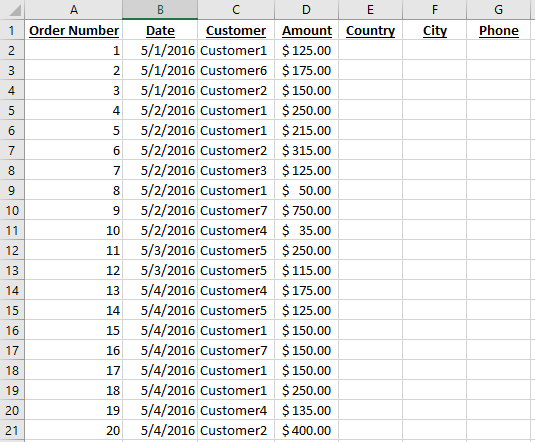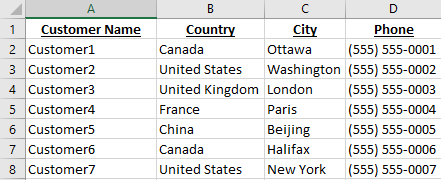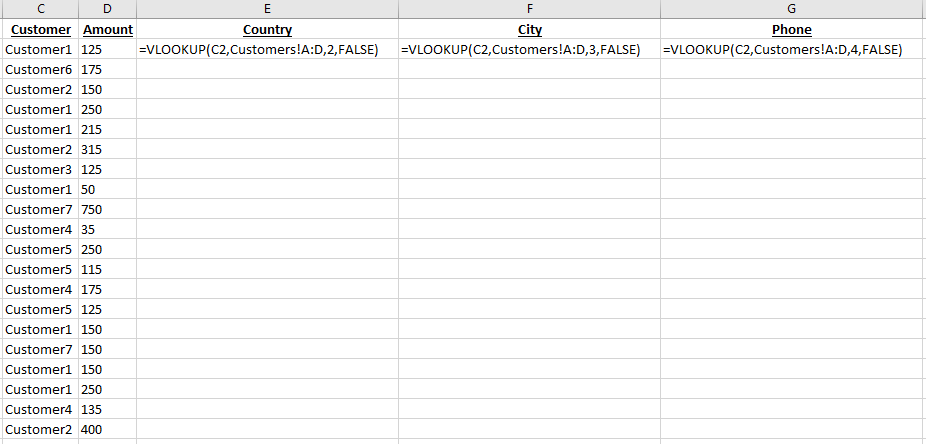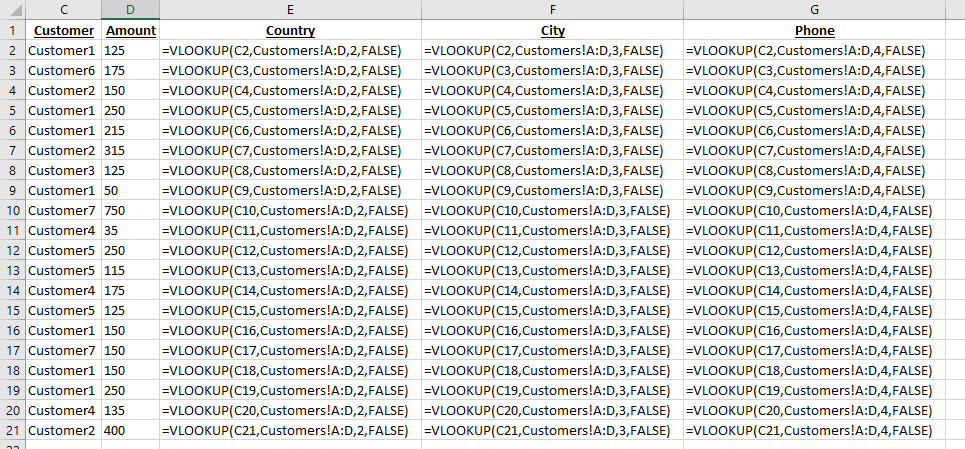www.ceporn.org cfnm party bitches get fucked. animetv amateur xxx fazendo fisting na escrava.## Excel Basic Formulas: Sum, Average, Count, Max, Min

Using Excel basic formulas can help simplify your life by doing sums, averages, counts, maxs, and mins of many numbers at the same time.

Efficient Analyst Excel Basic Functions Sum Average Count Min Max

## Excel Basic Formulas: Sum

The Sum formula is a great one to start with.  Excel formulas all start with an = sign, followed by the function name and a section in parentheses to provide ranges or arguments.  Start out by typing =sum(Sum requires a range of cells you want to add together.  You can type your range in, or you can select it using your mouse or input device.  In this case, we will select D2:D21.Next up you can close the parenthesis, although newer versions of Excel will auto close it for you.

## Excel Basic Formulas: Sum, Average, Min, Max, and Count

Using the same method, you can calculate the average, minimum value, maximum value, and count:Which if you were using the downloadable exercise workbook, will give you the following results:Here are some basic formulas, and what they do.

 Sum Adds all the cells together and gives you the total sum. Average Gives you the average of the selection you provide. Min Returns the smallest value from the selection you provide. Max Returns the largest value from the selection you provide. Count Counts how many cells have values in them.

• In the bottom right of the screen, newer versions of Excel provide you with a Sum, Average, and Count by default, and can be configured by right-clicking on it to provide minimum, maximum, and numerical count.## Creating an Excel Drop-down list using Data Validation List

Adding an Excel Drop-down list is a great way to make data entry faster, more efficient, and also more accurate.  There are a few ways they can be done, and in this article we show how to do them using the Data Validation lists.

Efficient Analyst Data Validation List Drop-down Exercise Workbook

## Procedure steps

To create an Excel drop-down list, select the cell where you would like the drop-down to appear, and open the Data ribbon.

In the Data ribbon, select Data Validation -> Data Validation…In the Data Validation dialog box, select List.Click the Select Source button.Type or select the range you would like to include as part of your Excel drop-down list.## Using the Excel VLOOKUP function

Using the Excel VLOOKUP function makes it easy to link two excel tables together, and can be a great time saver.

## Procedure steps

As an example of how the Excel Vlookup function works, let’s take a simple excel workbook with the following two tabs: Orders and Customers.The Orders tab contains an order number, date, customer name, and amount.We want to add the Country, City, and Phone number of each customer, which thankfully we have in the Customers tab.By using the Excel VLOOKUP function, we can quickly and efficiently fill in the Country, City, and Phone in the Orders tab.

Let’s go back to the Orders tab, and start typing the =vlookup( formula to see the tooltip provided by Microsoft:If you’ve never used the Excel vlookup function before, this provides absolutely no help.  But lets break it down in an easier way:

 Argument Description Example Example Code lookup_value The value which is in common between both tables In this case, Customer Name, more specifically, if we are trying to find the Country for the customer on line two (i.e. we are trying to fill cell E2), we would select cell C2 as the customer name. C2 tabble_array Where the information you are looking for is located. The first column should contain the common element, and the range should be large enough to include In this case, it would be the Customers tab, columns A through D. Customers!A:D col_index_num Based on the range you put in table_array, the col_index_num is the number of the column which contains the result you are looking for. For example, if you selected A:D as your range, and the common element is in column A, and the result is in column B, you would put 2 as your column number. Putting 3 would give you the result from column C, and putting 4 would give you the result from column D. However, if you select L:P as your range, then column 1 is L, column M is 2, column N is 3, etc. Because the country code is located in column B of the Customers tab, we will select 2 2 [range_lookup] Last and definitely not least, the range_lookup gives you a true or false option. False will only return an exact match, while True will try to look for a result which looks similar to what you are looking for. 99%+ of the time I find False to be the best option to use. For example, if you had an order for Customer8, because there is no Customer8 in the customers tab, using false would return #N/A meaning Excel could not find a match. This would let you know that you have to add Customer8 to the Customers tab. If you select true, that same Customer8 would return United States, because Customer8 looks similar to Customer7, which is in the US. false

We end up with the following formula: =VLOOKUP(C2,Customers!A:D,2,FALSE), where C2 is the Customer we are trying to match up, Customers!A:D contains the customer information we want to look into, 2 is the 2nd column from that range, and False because we only want exact matches.

Using the same method, we can create the formulas for F2 and G2 by changing the 2 to a 3 or a 4.  (I changed my view settings in Excel to show the formulas instead of the values, so you should see Canada, Ottawa, and (555) 555-0001 instead of the formulas)You can then select a formula and drag the little dot in the corner to fill in the other formulas very quickly:Which would give us the following formulas (again in formula view):Here is what you should see on your screen at the end.  By using the formula dragging feature, you can very quickly perform vlookups for thousands of records!